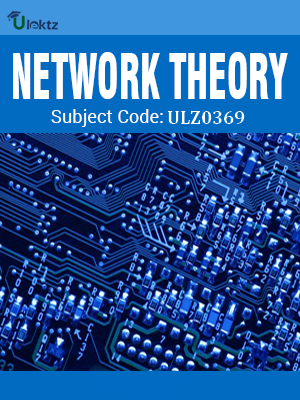•My WalletMy Order
•My Profile
•My Connections
•My Books
•My Videos
•My Tests
•My Calender
•My Messages
•My Shopping Cart
•My Orders
•Account Settings
•Help

# Book Details# Network Theory

 Course Code : ULZ0369 Author : uLektz University : General for All University Regulation : 2017 Categories : Computer Science Format :ePUB3 (DRM Protected) Type : eBook

FREE

Description :Network Theory of ULZ0369 covers the latest syllabus prescribed by General for All University for regulation 2017. Author: uLektz, Published by uLektz Learning Solutions Private Limited.

Note : No printed book. Only ebook. Access eBook using uLektz apps for Android, iOS and Windows Desktop PC.

##### Topics
###### Module- I Network Topology,Network Theorems & Coupled Circuits

1. 1 NETWORK TOPOLOGY: Graph of a network, Concept of tree, Incidence matrix, Tie-set matrix, Cut-set matrix, Formulation and solution of network equilibrium equations on loop and node basis

1.2 NETWORK THEOREMS & COUPLED CIRCUITS: Substitution theorem, Reciprocity theorem, Maximum power transfer theorem, Tellegen’s theorem, Millman’s theorem, Compensation theorem

1.3 Coupled Circuits, Dot Convention for representing coupled circuits, Coefficient of coupling, Band Width and Q-factor for series and parallel resonant circuits

###### Module- II Laplace Transform & Its Application,Two Port Network Functions & Responses

2.1 LAPLACE TRANSFORM & ITS APPLICATION: Introduction to Laplace Transform, Laplace transform of some basic functions, Laplace transform of periodic functions, Inverse Laplace transform, Application of Laplace transform: Circuit Analysis (Steady State and Transient)

2.2 TWO PORT NETWORK FUNCTIONS & RESPONSES: z, y, ABCD and h-parameters, Reciprocity and Symmetry, Interrelation of two-port parameters, Interconnection of two-port networks, Network Functions

2.3 Significance of Poles and Zeros, Restriction on location of Poles and Zeros, Time domain behaviour from Pole-Zero plots

###### Module- III Fourier Series & Its Application,Network Synthesis

3.1 FOURIER SERIES & ITS APPLICATION: Fourier series, Fourier analysis and evaluation of coefficients, Steady state response of network to periodic signals, Fourier transform and convergence, Fourier transform of some functions

3.2 Brief idea about network filters (Low pass, High pass, Band pass and Band elimination) and their frequency response.

3.3 NETWORK SYNTHESIS: Hurwitz polynomial, Properties of Hurwitz polynomial, Positive real functions and their properties, Concepts of network synthesis

3.4 Realization of simple R-L, R-C and L-C functions in Cauer-I, Cauer-II, Foster-I and Foster-II forms